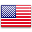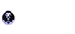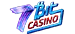# Blackjack Odds and Probability

Blackjack is a slightly deceptive game. Its simple rules of play may fool you into believing it is easy to master but if you delve deeper, you will quickly find this is a purely mathematical game that is all about odds and probabilities. Blackjack Hall of Fame inductees Edward Thorp and Julian Braun were among the first people to come to this realization in the 1960s. They ran millions of simulations on old IBM computers to refine the basic blackjack strategy Ed Thorp published in his Beat the Dealer book, which is now a classic in the blackjack canon.Best Online Casino Bonuses
\$
1. 350% up to
\$3500
Bonus
Play With
\$3600
Bankroll
2. 25% up to
\$9999925% up to \$99999
Up to 25% Rakeback on each bet! Rakeback is calculated as a return percentage on each of your bets, depending on the edge that the house has on you theoretically. Rakeback percentage is dependent on a player’s level. Levelling up is achieved through wagering with real money. Exclusive to slots. No rollover requirements. Total rakeback percentage for Bronze users can reach up to 14%. Total rakeback percentage for Silver users can reach up to 19%. Total rakeback percentage for Gold users can reach up to 24%. Total rakeback percentage for Platinum users is 25%.
Claim BonusVisit CryptoLeo Casino
Bonus
Play With
\$100099
Bankroll
3. 100% up to
\$300100% up to \$300
18+ New Customers only. Up to 1.5 BTC on first deposit and up to 5 BTC across first four deposits. 100 additional free spins. Up to €/\$ 300 first deposit bonus in fiat and up to €/\$5000 fiat welcome package. 40x wagering on bonuses. 14 day expiration time.
Claim BonusVisit 7Bit Casino
Bonus
Play With
\$400
Bankroll

If you truly want to win at this game, you need to gain a good understanding of what the odds and probabilities for every possible scenario at the table are and base your playing decisions on these odds. The following article aims at introducing you to the foundations of blackjack odds and probabilities. Toward the end, we have also included several charts that may prove to be useful.

## Understanding Blackjack ProbabilitiesMany people use “probability” and “odds” as two interchangeable terms but in fact, there is a pronounced difference between the two. While inherent in gambling, probability is, first and foremost, a separate branch of mathematics that deals with the likelihood of different events occurring. Probability permeates all aspects of our lives, from weather reports to statistics and playing at your local casino.

Probability is calculated on the basis of known data but cannot be used to predict exact outcomes, like the outcome of a hand in the game of blackjack, for example. It simply shows you the likelihood of an event happening, based on the knowledge of the number of desired outcomes and the number of all possible outcomes. You can use this knowledge to execute the best play at the blackjack table but it alone does not show you with absolute certainty which card the dealer will pull out next.

Statisticians use what is known as a “probability line” to represent the probabilities of events, which can be classified as certain, likely, unlikely, and altogether impossible. The further to the left an event is positioned on the probability line, the more unlikely it is to take place. Conversely, when an event is positioned further to the right of the line’s center, it has a higher likelihood of occurrence.

The probability of a given outcome happening is quite simple to calculate. All you have to do is divide the number of desired outcomes by the number of all possible outcomes. In the context of gambling, this translates into dividing the number of ways to win by the number of all possible outcomes.

### Independent vs. Dependent TrialsBefore we proceed with concrete examples, we would like to make a distinction between independent and dependent events (or trials in statistics). An independent event has no impact on another event’s probability of occurring (or not occurring). Such is the case with the dice tosses in the game of craps and roulette spins, where previous outcomes have no influence on the results of the trials that are to follow.

Here is an example of determining the probability of rolling a 2 with a six-sided dice. There is only one possible way for you to roll a 2 out of 6 possible outcomes. It follows that the likelihood of a 2 being rolled is 1/6 = 1.166 * 100 = 16.66%.

The probability of rolling a deuce with two dices is even slimmer because there are more permutations (36 to be precise) but there is only one two-dice combination that results in a 2. Respectively, the likelihood of this independent event occurring is 1/36 = 0.027 * 100 = 2.77%. No matter how many times you toss the dice, the probabilities of the tosses, or trials, will always remain the same.

With dependent events, on the other hand, previous trials influence the probabilities of the trials that are to follow. Unlike roulette and dice games, blackjack is a game of dependent trials where each card dealt changes the composition of the remaining deck and therefore, influences the likelihood of forming specific hands on the next rounds of play.

This phenomenon is best explained through examples, so let’s calculate the probability of drawing an Ace from a single deck of cards. Single-deck blackjack utilizes a full deck of 52 cards without any jokers where we have 13 card denominations of 4 different suits each, which is to say there are only 4 Aces out of 52 cards. Therefore, the likelihood of you pulling an Ace at random is P(Ace) = 4/52 = 0.0769 * 100 = 7.69%.

### Another Example – Hard 16 vs. Dealer 10 (No Surrender)Let’s demonstrate how probabilities in blackjack work when more cards have left the deck. We assume you start a fresh round in a no-hole-card game after the single deck has been reshuffled. You are dealt Q-6 against a dealer K but surrender is unavailable, in which case you are forced to hit your hard 16. What is the likelihood of improving your total on the next hit?

We are left with 49 cards since 3 cards have been removed from the deck already. The following cards can help you improve your 16: an Ace for a total of 17 (your Ace will be counted as 1 in this case since otherwise it will bust your hand), a deuce for a total of 18, a 3 for a total of 19, a 4 for a total of 20, and a 5 for the best possible outcome of 21.

Thus, you have 20 cards that can help you out of 49. The probability of drawing a “good” card is 20/49 = 0.408 * 100 = 40.8%. Conversely, the likelihood of you busting by pulling one of the 29 “bad” cardsis 29/49 = 0.591 * 100 = 59.1%.

### The Probability of Obtaining a BlackjackNaturals are the strongest hands you can obtain in the game of 21. Not only it is impossible to lose with a natural (in the worst-case scenario you will push with the dealer) but you get a little extra in terms of profits since blackjacks return 1.5 times your original bet (on condition you are not foolish enough to play 6-to-5 games). Because of this, it is important for you to gain a proper understanding of the probabilities of getting blackjacks.

Knowing the number of decks in play, you can easily determine the likelihood of receiving a natural after the reshuffle. For the purpose, you must multiply the probability of pulling an Ace by the probability of pulling ten-valued cards like 10, J, Q, and K (there are four of each in a single pack for a total of 16). It is also necessary to multiply the result by 2 because there are two possible permutations of cards in a hand of blackjack, for example A-Q and Q-A, K-A and A-K, and so on.

The probability of drawing an Ace is 4/52 while that of pulling one of the ten-value cards is 16/51. The number of cards has dropped to 51 in the second case to account for the Ace that has been removed from the deck. Therefore, we calculate the probability of getting dealt a blackjack in the following way: P (Ace) * P (Ten-Value Card) * 2 = (4/52) * (16/51) * 2 = 0.0482 * 100 = 4.82%.

The likelihood of getting naturals decreases as more decks are introduced into the game, which, inturn, slightly increases the advantage the casino has over you. This often sounds antithetical to inexperienced players who reason it should be the other way around because there are more Aces and ten-value cards when multiple decks are used.

## Converting Probability into OddsOdds are different than probability in that they show us the ratio between the number of desired outcomes occurring and the number of ways in which the desired outcome will not occur. In the context of gambling, this corresponds to the ratio between winning and losing outcomes. Unlike probability, the odds are normally expressed as fractions instead of as percentages.

Here are a couple of examples so you can get a firmer grasp on how odds work. Let’s suppose you are interested to know the odds of you hitting number 9 in single-zero roulette where there are 37 numbers on the wheel in total. There is only one number that wins, so it follows there are 36 ways for you to lose. Respectively, the odds for you succeeding are 1 to 36, or 1/36. This corresponds to implied probability of 2.70% which weirdly enough coincides with the advantage of the casino in this game.

Let’s use another example with a single-deck blackjack game. What are the odds of you pulling the Queen of Spades from the 52-card pack? There is only one Queen of Spades in the deck opposed to 51 cards of different suits and denominations, so the odds of you drawing this card are 1 to 51 or 1/51.

In gambling, odds are normally expressed in reverse showing you the chances “against” an outcome occurring, like so: 51 to 1 and 36 to 1. You can convert the implied probability into odds with the following formula: (100/P) – 1 where P stands for probability.

## The Probability of the Dealer Busting and the Effect of Cards’ RemovalIn blackjack, the odds and probabilities fluctuate with each card that leaves the deck or shoe. This is so because small cards 2 through 6 favor the dealer, whereas high cards 10, J, Q, K, and A favor the player. Cards 7 through 9 are neutral because they favor neither the player nor the dealer.

The dealer has higher chances of exceeding 21 when they start their hand with small cards like 4, 5, and 6. The player’s advantage increases when the dealer exposes one of these cards. Respectively, the player’s advantage begins to drop when high cards are removed from the deck. Examine the table below for more information on the dealer’s probability of busting with individual upcards.

The Dealer’s Exposed Card The Probability of the Dealer Busting with This Card in S17 Games The Player Advantage against a Dealer Showing the Card
Ace 11.65% -16.00%
2 35.30% 9.80%
3 37.56% 13.40%
4 40.28% 18.00%
5 42.89% 23.20%
6 42.08% 23.90%
7 25.99% 14.30%
8 23.86% 5.40%
9 23.34% -4.30%
10, J, Q, K 21.43% -16.90%

Blackjack is the only casino game where cards “have a memory” since your chances of winning change each time a card is removed from the deck. In fact, this is the basic premise of card counting which we discuss further on in this guide.

When the composition of the deck or shoe is such that ten-value cards and Aces outnumber small card, the player holds an advantage over the dealer. It is the other way around when there are more small cards left to be played. The table below shows you how the cards of different ranks impact your chances of winning:

Card That Leaves the Deck Impact of the Card’s Removal on Players’ Chances of Winning
A -0.59%
2 0.40%
3 0.43%
4 0.52%
5 0.67%
6 0.45%
7 0.30%
8 0.01%
9 -0.15
10, J, Q, K -0.51